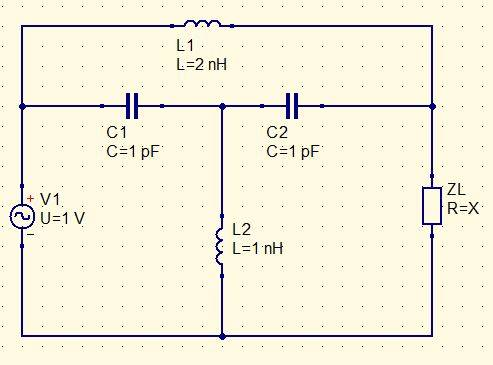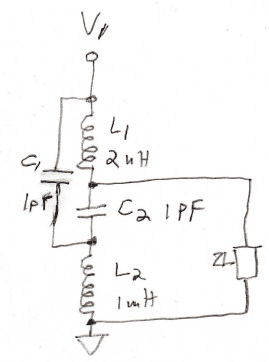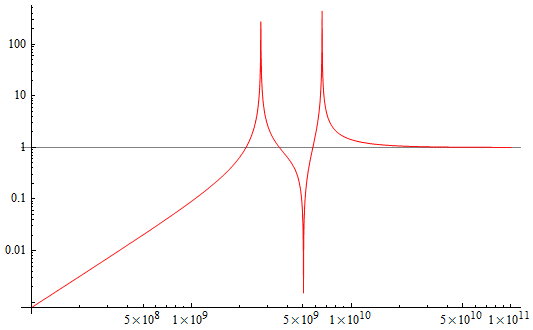# Applying symmetry to an asymmetrical circuit

• Engineering
Thread moved from the technical forums to the schoolwork forums
Homework Statement:
.
Relevant Equations:
.
Hello everyone,
I need to solve a problem applying symmetry and superposition theorem but the problem is that the circuit is almost symetrical but has little differences in both sides (the source in the left side and the unknown impedance in the right side). I couldn't solve the problem using only Kirchoff's laws.
An example would be:I know I can separate L1 in two inductances in series and L2 in two inductances in paralel but I don't know what to do with ZL.
The problem asks to determine the potential difference in ZL and the value of ZL when the potential in L2 is 0.
I would thank any help no matter how small

•Delta2

DaveE
Gold Member
You can start with some deductions about the currents in each branch when the voltage across L2 is 0. KCL will be helpful.

DaveE
Gold Member
I couldn't solve the problem using only Kirchoff's laws.
I guarantee that this circuit can be solve with Kirchhoff's laws. I'm not clear about if that is allowed here, but it is absolutely something that you will need to know in the future.

I haven't thought too hard about this solution, but I think you have to use Kirchhoff's laws to solve the more general question posed. But then maybe I've missed some trick to simplify things.

Superposition applies to circuits with multiple independent sources, I don't see how it's useful here. In particular, it does not apply to changing circuit elements or values (like ZL) that aren't independent current or voltage sources.

DaveE
Gold Member
If you're familiar with simple networks, you could model the inductor & capacitor network using something like Z-parameters and then add the source and load to find a solution. That network has obvious symmetry which will show up in the Z matrix. But I doubt that is really different than using Kirchhoff.

BTW, this network is called a "bridged-tee", "bridge t", or similar by EEs. It very often (but not always) has symmetric values.

Thank you very much for your answer. I’ll try solving the problem in this way again, I think I should be able taking into account the voltage across L2.

The only problem is that our teacher adviced us to use superposition and symmetry. But maybe is complicating unnecessarily things.

Baluncore
Welcome to PF.

The only problem is that our teacher adviced us to use superposition and symmetry.
This is a trick question.

C1 in series with C2 is a centre tapped capacitor,
that with L1 makes a centre tapped resonator.
Think of L1+C1+C2 as a centre tapped transformer.

Under what condition will V(L2) = zero?

DaveE
Gold Member
Welcome to PF.

This is a trick question.

C1 in series with C2 is a centre tapped capacitor,
that with L1 makes a centre tapped resonator.
Think of L1+C1+C2 as a centre tapped transformer.

Under what condition will V(L2) = zero?
Yes, but, will this help a student that is having problems solving a simple network with Kirchhoff's laws?
Do you really think referring to resonators and center-tapped transformers will be helpful?

•Delta2
DaveE
Gold Member
Thank you very much for your answer. I’ll try solving the problem in this way again, I think I should be able taking into account the voltage across L2.

The only problem is that our teacher adviced us to use superposition and symmetry. But maybe is complicating unnecessarily things.
What is the current through L2 when the voltage (potential) across it is zero?
What does that tell you about the current through the capacitors?
Then, what does that tell you about the voltage across the capacitors?
Then, what would KVL tell you about the voltages in the capacitor-source-load loop?

Baluncore
Yes, but, will this help a student that is having problems solving a simple network with Kirchhoff's laws?
Do you really think referring to resonators and center-tapped transformers will be helpful?
Yes. The centre-tapped transformer reference was a clue.

Looking for and recognising simple (lossless) resonators is part of the exercise. It resolves the whole of the problem without the massive error prone exercise in KL, followed by cancellation.

The resonator, with L2, is a symmetrical circuit. Apart from that there is only the source and a load.

The zero voltage across L2 is the biggest clue that could ever be given.
The source and load must be very simply related.

I await a solution via KL.
I will be interested to see if it confirms the answer.

DaveE
Gold Member
The zero voltage across L2 is the biggest clue that could ever be given.
Yes, but it is also the problem statement. Without that condition the solution is a mess. It allows us to solve a much simpler network, with some known values to allow solving one thing at a time. Much better than a 6D matrix inversion, even if it has a lot of 0's.

The Electrician
Gold Member
Thank you very much for your answer. I’ll try solving the problem in this way again, I think I should be able taking into account the voltage across L2.

The only problem is that our teacher adviced us to use superposition and symmetry. But maybe is complicating unnecessarily things.
Did your teacher "advise" you, or "require" you to use superposition and symmetry?

The Electrician
Gold Member
Homework Statement:: .
Relevant Equations:: .

Hello everyone,
I need to solve a problem applying symmetry and superposition theorem but the problem is that the circuit is almost symetrical but has little differences in both sides (the source in the left side and the unknown impedance in the right side). I couldn't solve the problem using only Kirchoff's laws.
An example would be:
View attachment 287460
I know I can separate L1 in two inductances in series and L2 in two inductances in paralel but I don't know what to do with ZL.
The problem asks to determine the potential difference in ZL and the value of ZL when the potential in L2 is 0.
I would thank any help no matter how small
sergiohjm, the schematic you posted here appears to have been drawn by you, and not to be the actual schematic supplied with the problem statement. Is this true?

Please check the values of L1 and L2 because they are not in the ratio usually found in a bridged tee notch network--I think they may be switched.

The Electrician
Gold Member
Yes. The centre-tapped transformer reference was a clue.

Looking for and recognising simple (lossless) resonators is part of the exercise. It resolves the whole of the problem without the massive error prone exercise in KL, followed by cancellation.

The resonator, with L2, is a symmetrical circuit. Apart from that there is only the source and a load.

The zero voltage across L2 is the biggest clue that could ever be given.
The source and load must be very simply related.

I await a solution via KL.
I will be interested to see if it confirms the answer.
Are you sure that it is possible for the voltage across L2 to be zero in the given circuit if ZL is a pure resistance with a finite value?

Baluncore
Are you sure that it is possible for the voltage across L2 to be zero in the given circuit if ZL is a pure resistance with a finite value?
Where is it specified that ZL must be a pure resistance with a finite value?

The Electrician
Gold Member
Where is it specified that ZL must be a pure resistance with a finite value?
The problem asks for the value of ZL. On the schematic under the ZL designator it says "R=X". The "X" is a common designator for an unknown, and the "R" is for resistor. So I took "R=X" to mean that ZL is a resistor of unknown value, presumably finite. The problem gives us no other clue as to the nature of ZL. I certainly wouldn't stake my life on this. How do you interpret the "R=X"?

DaveE
Gold Member
The problem asks for the value of ZL. On the schematic under the ZL designator it says "R=X". The "X" is a common designator for an unknown, and the "R" is for resistor. So I took "R=X" to mean that ZL is a resistor of unknown value, presumably finite. The problem gives us no other clue as to the nature of ZL. I certainly wouldn't stake my life on this. How do you interpret the "R=X"?

The Electrician
Gold Member
To say that the voltage across L2 is zero means that the top of L2 is at ground potential, which apparently allows the "center tapped transformer" to work its magic. It's tempting to think that the presence of L2 makes the "center tapped transformer" work. But if the voltage across L2 is zero, then L2 can be removed without changing the circuit behavior, and what will provide the "ground" at the center tap so it will work?

Baluncore
How do you interpret the "R=X"?
I see the text there as a mistranslation from the original question.

R is resistance = the real part of impedance.
X is reactance = the imaginary part of impedance.
Z = R +j X, the complex impedance.

It could mean that the real and imaginary components are equal, but I discount that on the grounds that it does not appear in the problem statement, and the OP submitted this as an example of a type of problem.

DaveE
Gold Member
what will provide the "ground" at the center tap so it will work?
That is provided by the artificial constraint that ZL WILL make that condition happen. This isn't a question about the network, it is about the network with a very special value of ZL.

DaveE
Gold Member
This network, without the load, is comprised entirely of reactive elements. What are the chances that a resistive load provides the required balance of no voltage at L2? Hint: yes but only at one frequency, where, BTW, other problems occur.

Seriously, this is not a difficult algebraic solution once you look at the consequences of having no voltage at L2. As we have said, the network gets much simpler in that case. At some point maybe I'll post the solution, but I'm waiting for the OP to learn stuff first.

Baluncore
An example would be: ...
Maybe you should post the original problem exactly as it was given to you.

The Electrician
Gold Member
Thank you very much for your answer. I’ll try solving the problem in this way again, I think I should be able taking into account the voltage across L2.

The only problem is that our teacher adviced us to use superposition and symmetry. But maybe is complicating unnecessarily things.
The circuit can be redrawn like this:If ZL is not present all we have is a voltage divider with L2 as the bottom impedance, and the combination of L1, C1, and C2 as the top impedance. It's almost trivial to develop an expression for the output of the divider (the voltage across L2) when V1 (1 volt RMS) is the input to the divider. If we plot that expression (with ZL absent) vs. frequency we get something like this:It looks like the voltage across L2 might be going to zero around 5 GHz. It will be more difficult to derive an expression for the L2 voltage when ZL is present. I leave it up to the OP to determine if in fact the L2 voltage goes all the way down to zero for some ZL.

The Electrician
Gold Member

The Electrician
Gold Member
sergiohjm replied once in this thread, in post #5. He has not responded since to several questions and offers of help if he would post his work. I think he has left, not to be heard from again. It would not be unreasonable to go ahead and post solutions now. Baluncore and DaveE, what do you have to show us?

berkeman
Mentor
It would not be unreasonable to go ahead and post solutions now.
Looks like he joined and was last seen both on last Thursday. Let's give him a week to come back before we share any solutions. Thanks.

sergiohjm
A PF Quark

Joined
Thursday, 11:39 AM

Last seen
Thursday, 12:54 PM